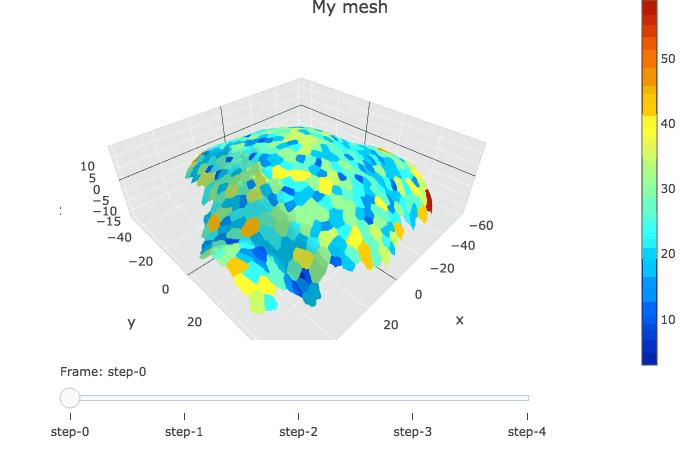The goal of this vignette is to highlight the power of R and Plotly regarding the visualisation of time series.

## Librairies

library(colorRamps)
library(dplyr)
library(plotly)
library(purrr)
library(mgx2r)

library(cellviz3d)

To install {cellviz3d}:

# devtools::install_github("marionlouveaux/cellviz3d")
library(cellviz3d)

## Data (all meshes from one individual)

Some .ply demonstration data coming from my PhD thesis are attached to this package and used here in the vignette. This dataset is a timelapse recording of the development of a WT shoot apical meristem expressing a membrane marker. I took one 3D stack every 12h and have 5 timepoints in total. Here I load the .ply and cell graph .ply for all the timepoints of this timelapse recording.

ply.dir <- system.file("extdata", "full/normalMesh/", package = "mgx2r")

mesh.all <- map(list.files(ply.dir, recursive = TRUE, full.names = TRUE),
~ read_mgxPly(file = .x, ShowSpecimen = FALSE))

graph.dir <- system.file("extdata", "full/cellGraph/", package = "mgx2r")

cellGraph.all <- map(list.files(graph.dir, recursive = TRUE, full.names = TRUE), ~read_mgxCellGraph(fileCellGraph = .x, header_max = 30))

## Plotly with slider

In plotly, the slider option allows to visualise several graphs linked by a time variable.

meshColors.all <- list(NULL, NULL, NULL, NULL, NULL)

plotlyMesh_all(meshExample = mesh.all,
graphExample = cellGraph.all,
meshColors = meshColors.all,
display = 'heatmap')